# Simple harmonic motion Questions and Answers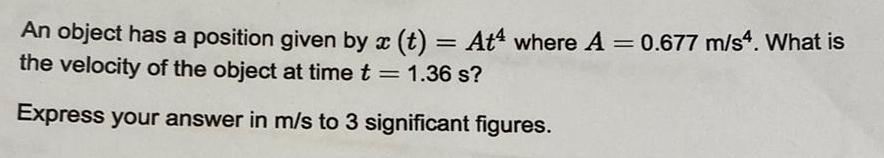Physics
Simple harmonic motion
An object has a position given by x (t) = At where A = 0.677 m/s4. What is the velocity of the object at time t = 1.36 s? Express your answer in m/s to 3 significant figures.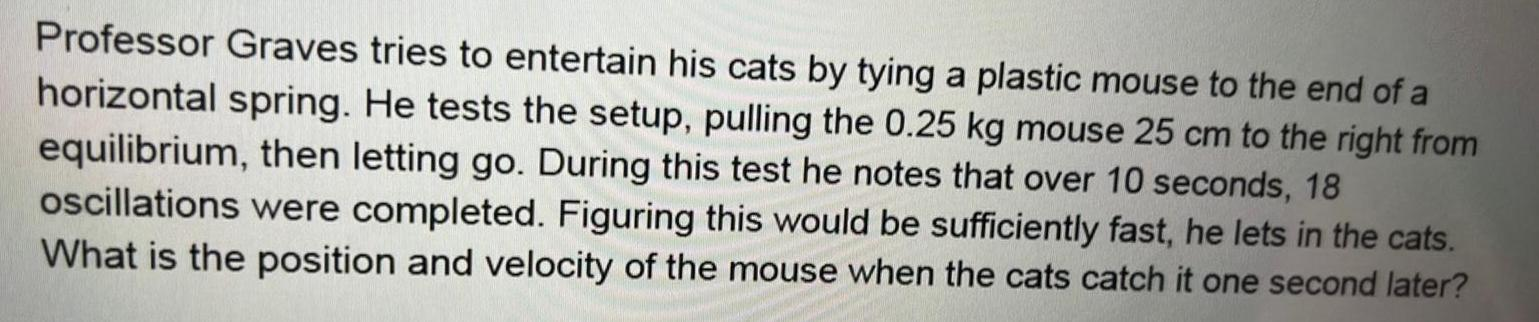Physics
Simple harmonic motion
Professor Graves tries to entertain his cats by tying a plastic mouse to the end of a horizontal spring. He tests the setup, pulling the 0.25 kg mouse 25 cm to the right from equilibrium, then letting go. During this test he notes that over 10 seconds, 18 oscillations were completed. Figuring this would be sufficiently fast, he lets in the cats. What is the position and velocity of the mouse when the cats catch it one second later?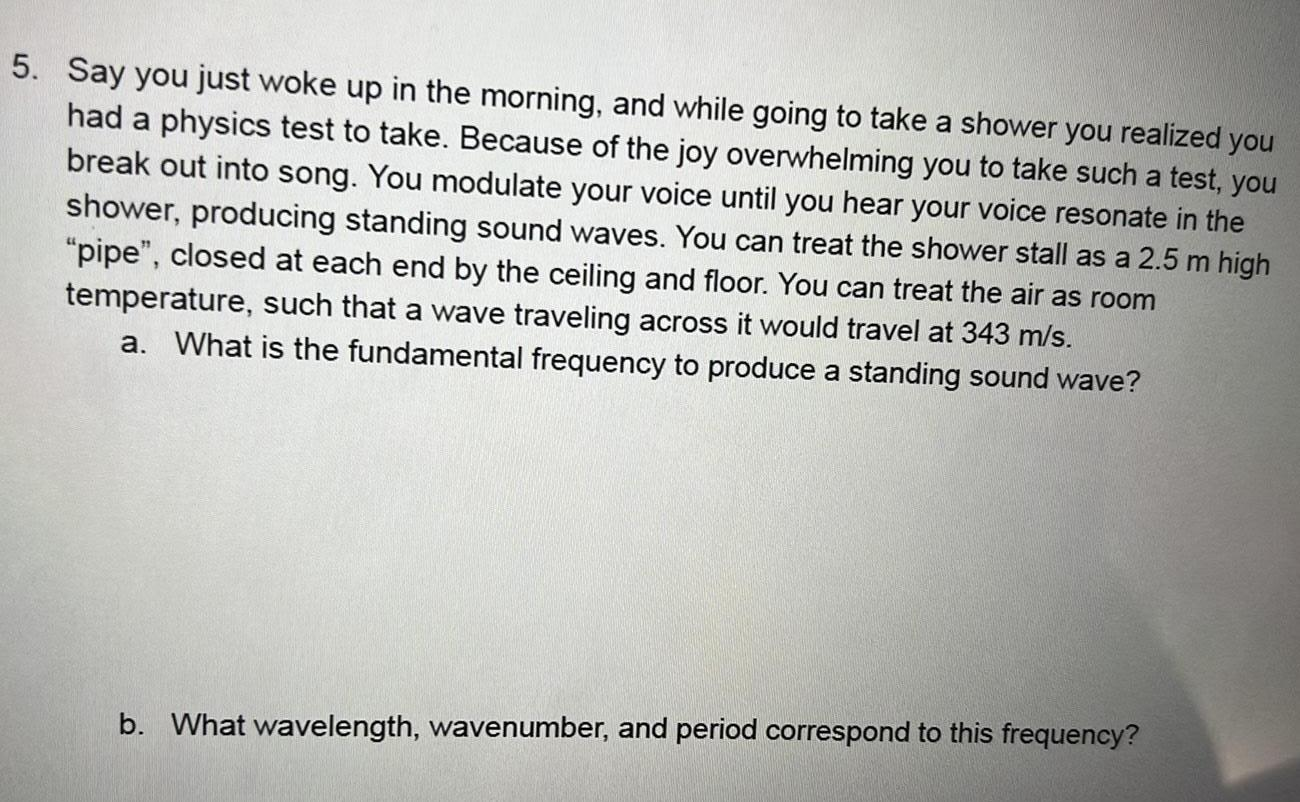Physics
Simple harmonic motion
Say you just woke up in the morning, and while going to take a shower you realized you had a physics test to take. Because of the joy overwhelming you to take such a test, you break out into song. You modulate your voice until you hear your voice resonate in the shower, producing standing sound waves. You can treat the shower stall as a 2.5 m high "pipe", closed at each end by the ceiling and floor. You can treat the air as room temperature, such that a wave traveling across it would travel at 343 m/s. a. What is the fundamental frequency to produce a standing sound wave? b. What wavelength, wavenumber, and period correspond to this frequency?Physics
Simple harmonic motion
If a simple harmonic oscillator has got a displacement of 0.04 m and acceleration equal to 3.06 m/s² at any time, what is the angular frequency of the oscillator in radian/sec? Round your answer to 2 decimal places.Physics
Simple harmonic motion
For the spring/mass system with an external force of the form Fext = sin(bt), what needs to be the case to see the exact phenomenon of beats? No damping, and a natural frequency in yc of exactly b. No damping, and a natural frequency in yc close to b. Light damping, and a natural frequency in yc of exactly b. Light damping, and a natural frequency in yc close to b.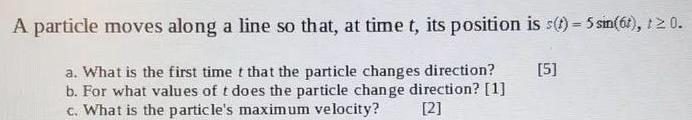Physics
Simple harmonic motion
A particle moves along a line so that, at time t, its position is s(t) = 5 sin(6), t>0. a. What is the first time t that the particle changes direction? b. For what values of t does the particle change direction?  c. What is the particle's maximum velocity?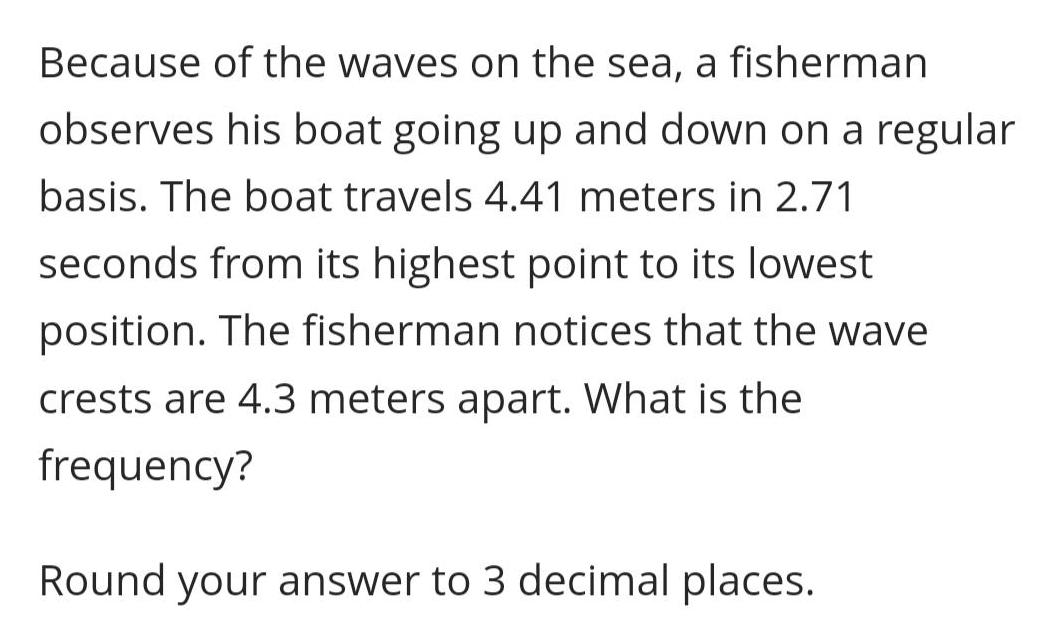Physics
Simple harmonic motion
Because of the waves on the sea, a fisherman observes his boat going up and down on a regular basis. The boat travels 4.41 meters in 2.71 seconds from its highest point to its lowest position. The fisherman notices that the wave crests are 4.3 meters apart. What is the frequency? Round your answer to 3 decimal places.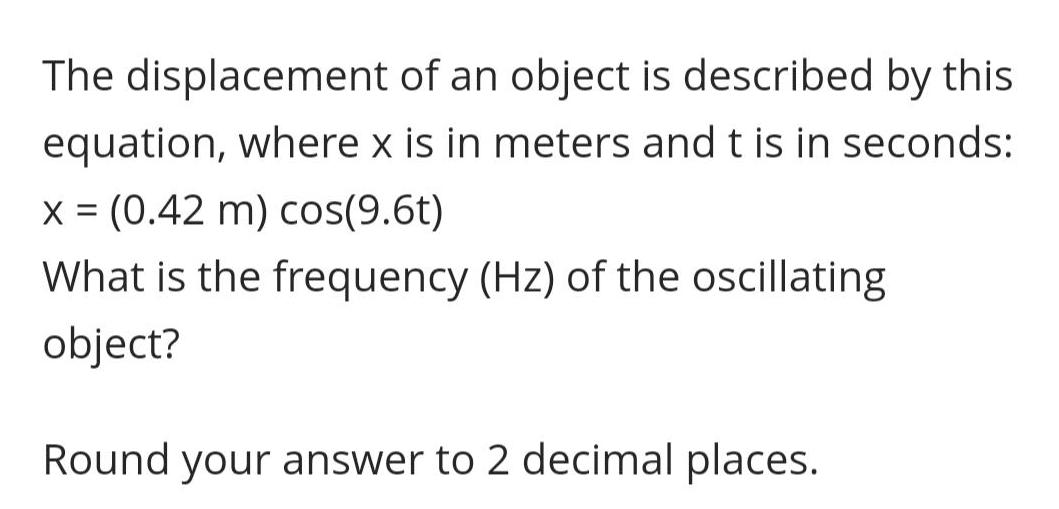Physics
Simple harmonic motion
The displacement of an object is described by this equation, where x is in meters and t is in seconds: x = (0.42 m) cos(9.6t) What is the frequency (Hz) of the oscillating object? Round your answer to 2 decimal places.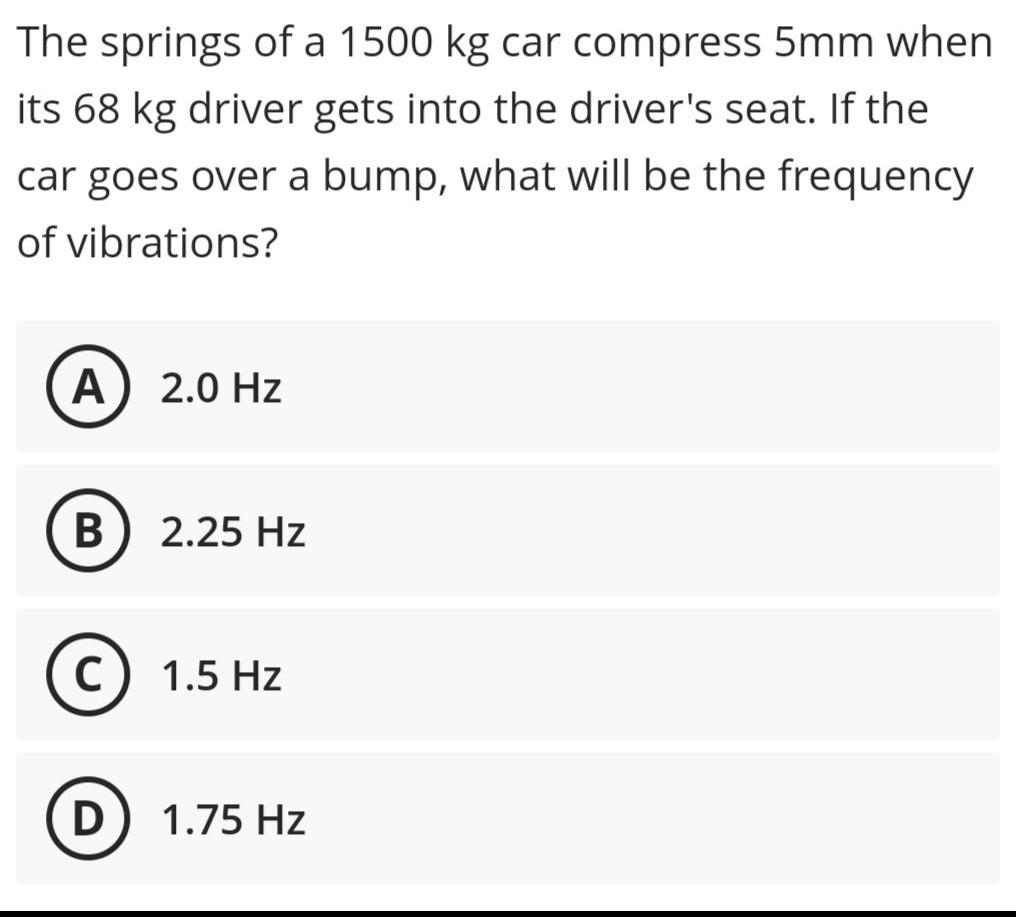Physics
Simple harmonic motion
The springs of a 1500 kg car compress 5mm when its 68 kg driver gets into the driver's seat. If the car goes over a bump, what will be the frequency of vibrations? A) 2.0 Hz B2.25 Hz C) 1.5 Hz D) 1.75 Hz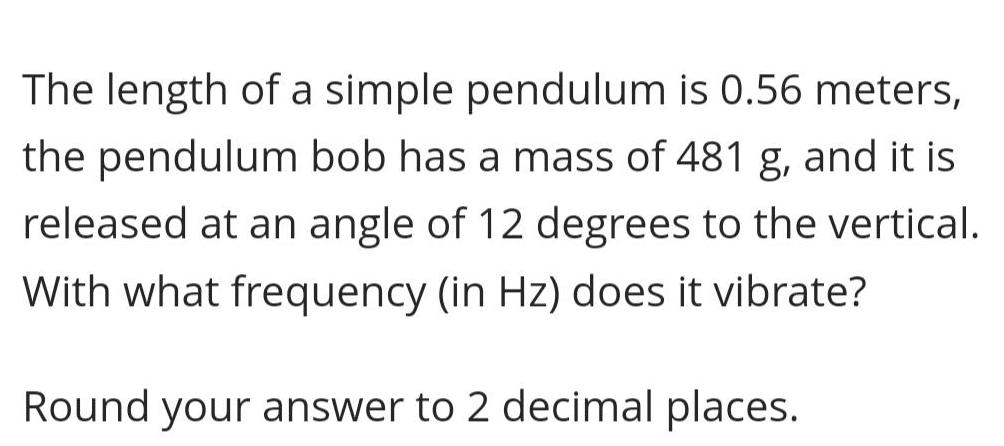Physics
Simple harmonic motion
The length of a simple pendulum is 0.56 meters, the pendulum bob has a mass of 481 g, and it is released at an angle of 12 degrees to the vertical. With what frequency (in Hz) does it vibrate? Round your answer to 2 decimal places.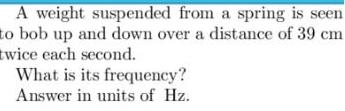Physics
Simple harmonic motion
A weight suspended from a spring is seen to bob up and down over a distance of 39 cm twice each second. What is its frequency? Answer in units of Hz.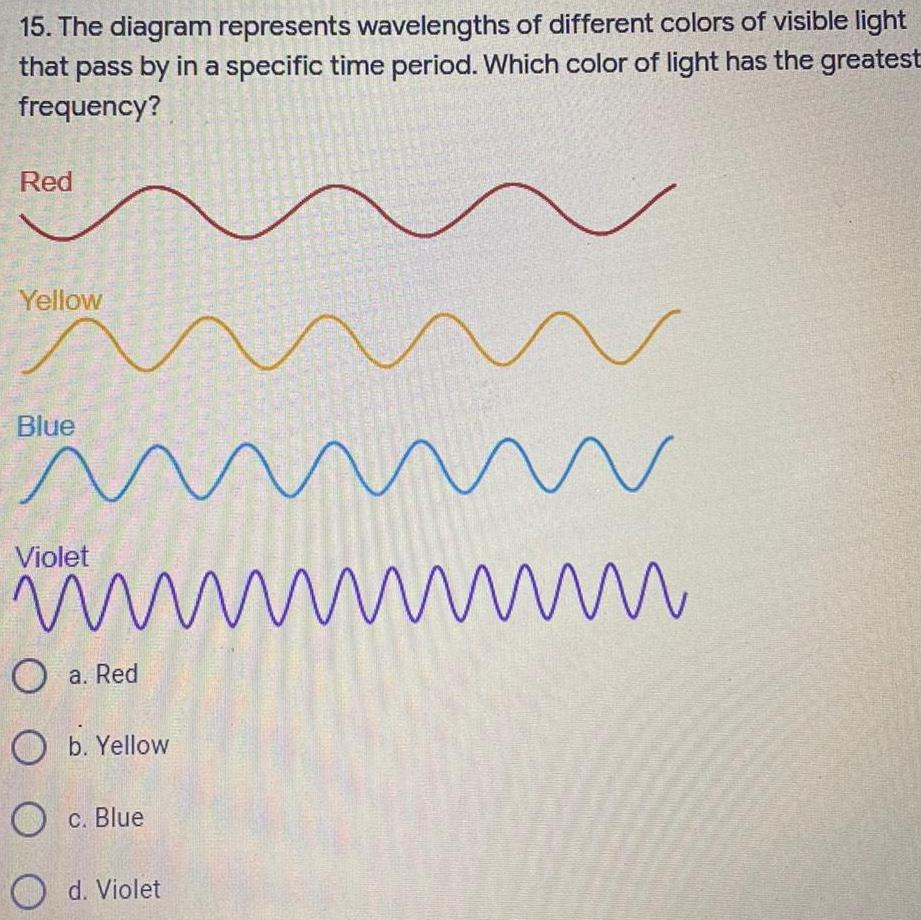Physics
Simple harmonic motion
15. The diagram represents wavelengths of different colors of visible light that pass by in a specific time period. Which color of light has the greatest frequency? Red Yellow Blue Violet a. Red b. Yellow c. Blue d. Violet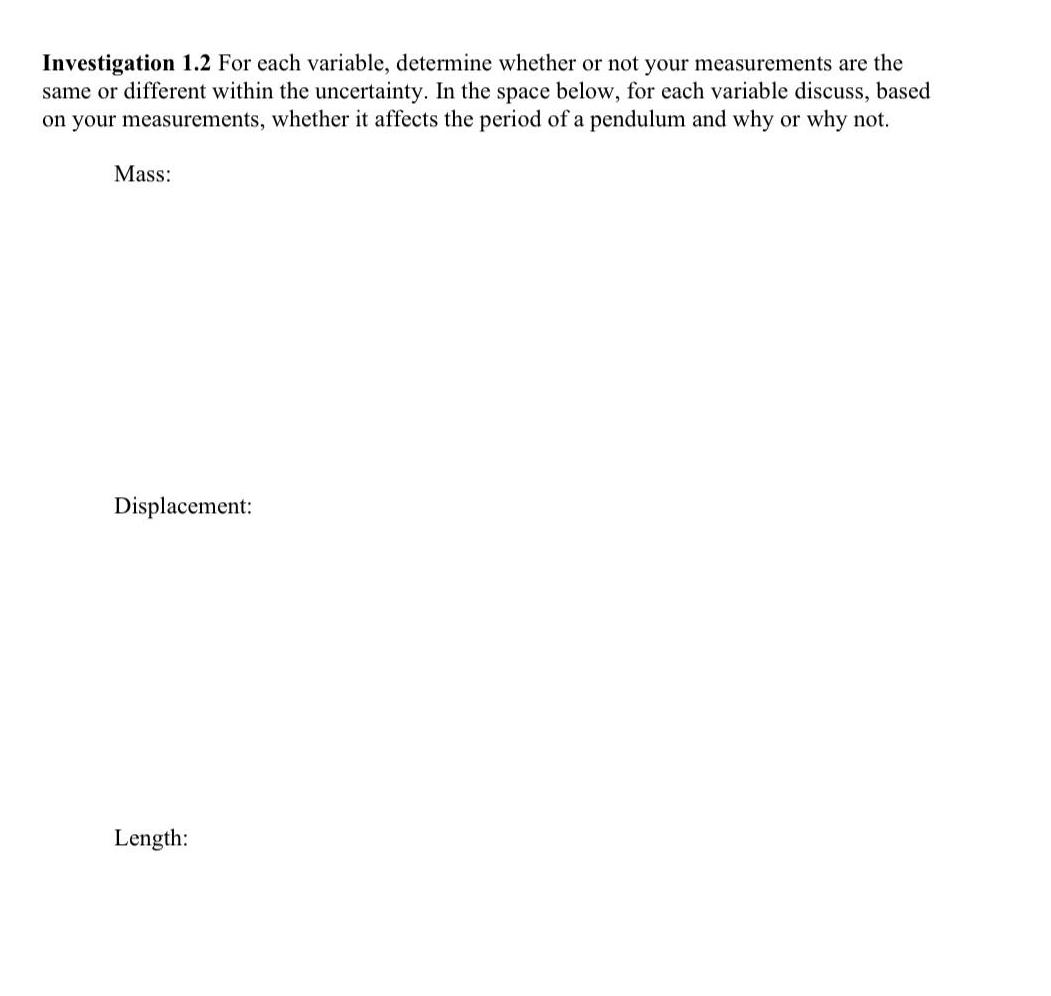Physics
Simple harmonic motion
Investigation 1.2 For each variable, determine whether or not your measurements are the same or different within the uncertainty. In the space below, for each variable discuss, based on your measurements, whether it affects the period of a pendulum and why or why not. Mass: Displacement: Length:Physics
Simple harmonic motion
A mass hanging from a spring undergoes vertical simple harmonic motion. Where in the motion is the magnitude of the net force equal to zero? At the highest point in the oscillation. At the lowest point in the oscillation. At the point half-way between the highest point and the lowest point. At the point where the spring is unstretched. At any point the net force is not zero. Where in the motion is the velocity equal to zero? Check all that apply. At the highest point in the oscillation. At the point half-way between the highest point and the lowest point. At the point where the spring is unstretched. At the lowest point in the oscillation.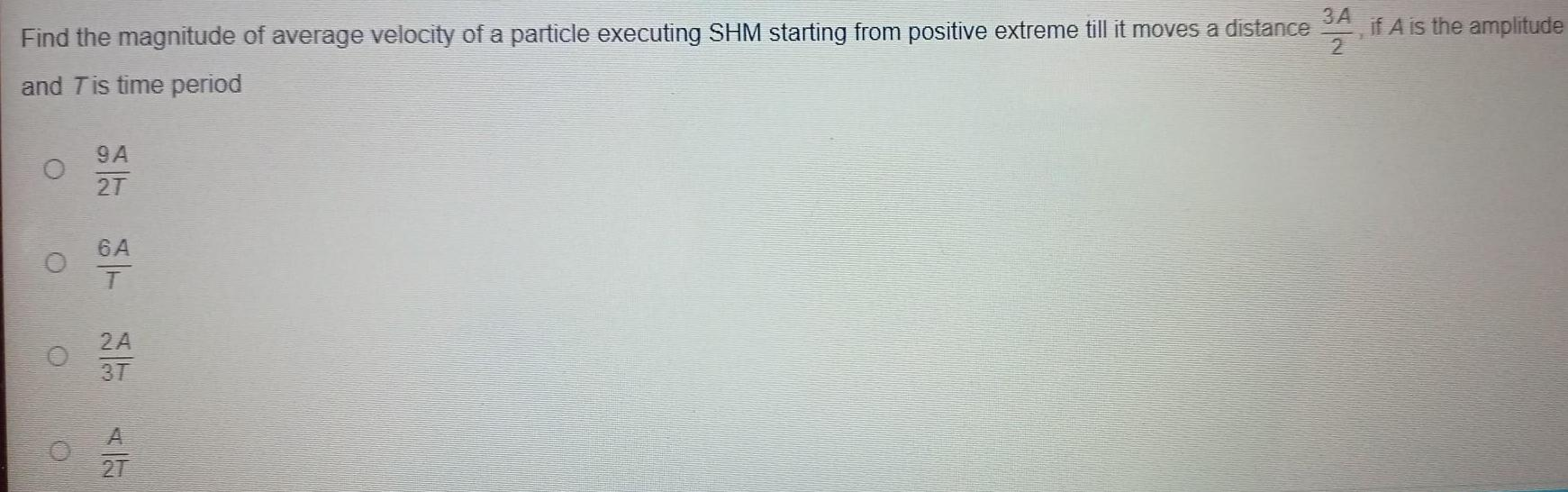Physics
Simple harmonic motion
Find the magnitude of average velocity of a particle executing SHM starting from positive extreme till it moves a distance 3A/2 if A is the amplitude and T is time period 9A/2T 6A/T 2A/3T A/27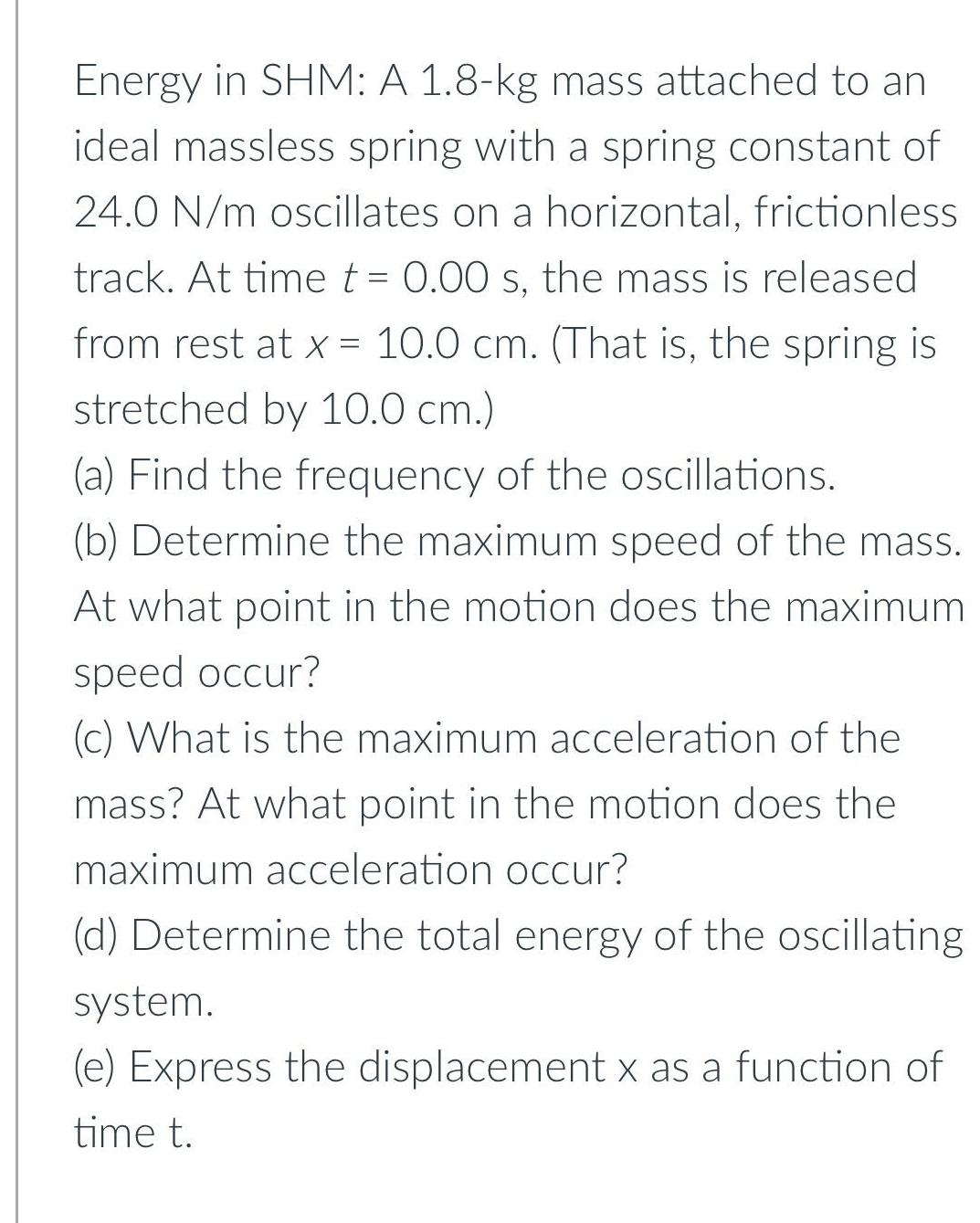Physics
Simple harmonic motion
Energy in SHM: A 1.8-kg mass attached to an ideal massless spring with a spring constant of 24.0 N/m oscillates on a horizontal, frictionless track. At time t = 0.00 s, the mass is released from rest at x = 10.0 cm. (That is, the spring is stretched by 10.0 cm.) (a) Find the frequency of the oscillations. (b) Determine the maximum speed of the mass. At what point in the motion does the maximum speed occur? (c) What is the maximum acceleration of the mass? At what point in the motion does the maximum acceleration occur? (d) Determine the total energy of the oscillating system. (e) Express the displacement x as a function of time t.